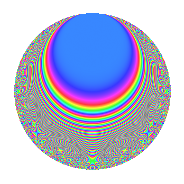# Properties

 Label 804.2.e.aLevel 804 Weight 2 Character orbit 804.e Analytic conductor 6.420 Analytic rank 0 Dimension 34 CM No

# Related objects

## Newspace parameters

 Level: $$N$$ = $$804 = 2^{2} \cdot 3 \cdot 67$$ Weight: $$k$$ = $$2$$ Character orbit: $$[\chi]$$ = 804.e (of order $$2$$ and degree $$1$$)

## Newform invariants

 Self dual: No Analytic conductor: $$6.41997232251$$ Analytic rank: $$0$$ Dimension: $$34$$ Sato-Tate group: $\mathrm{SU}(2)[C_{2}]$

## $q$-expansion

The dimension is sufficiently large that we do not compute an algebraic $$q$$-expansion, but we have computed the trace expansion.

 $$\operatorname{Tr}(f)(q) =$$ $$34q - 34q^{3} + 2q^{4} - 4q^{7} + 6q^{8} + 34q^{9} + O(q^{10})$$ $$\operatorname{Tr}(f)(q) =$$ $$34q - 34q^{3} + 2q^{4} - 4q^{7} + 6q^{8} + 34q^{9} - 6q^{10} - 2q^{12} - 4q^{14} + 2q^{16} + 12q^{20} + 4q^{21} - 8q^{22} - 6q^{24} - 34q^{25} + 10q^{26} - 34q^{27} + 8q^{28} - 16q^{29} + 6q^{30} + 4q^{31} + 2q^{36} + 12q^{37} - 26q^{38} - 18q^{40} + 4q^{42} + 4q^{43} - 26q^{44} + 4q^{46} - 2q^{48} + 46q^{49} + 18q^{50} - 32q^{52} + 14q^{56} - 4q^{58} - 12q^{60} - 2q^{62} - 4q^{63} + 26q^{64} + 8q^{66} + 18q^{67} - 34q^{68} - 56q^{70} + 6q^{72} + 12q^{73} - 22q^{74} + 34q^{75} - 32q^{76} - 8q^{77} - 10q^{78} + 12q^{79} + 2q^{80} + 34q^{81} + 26q^{82} - 8q^{84} + 6q^{86} + 16q^{87} - 28q^{88} - 6q^{90} - 46q^{92} - 4q^{93} - 32q^{94} + 40q^{95} + 40q^{98} + O(q^{100})$$

## Embeddings

For each embedding $$\iota_m$$ of the coefficient field, the values $$\iota_m(a_n)$$ are shown below.

For more information on an embedded modular form you can click on its label.

Label $$a_{2}$$ $$a_{3}$$ $$a_{4}$$ $$a_{5}$$ $$a_{6}$$ $$a_{7}$$ $$a_{8}$$ $$a_{9}$$ $$a_{10}$$
535.1 −1.41091 0.0966035i −1.00000 1.98134 + 0.272598i 2.83991i 1.41091 + 0.0966035i 0.830707 −2.76915 0.576015i 1.00000 −0.274345 + 4.00685i
535.2 −1.41091 + 0.0966035i −1.00000 1.98134 0.272598i 2.83991i 1.41091 0.0966035i 0.830707 −2.76915 + 0.576015i 1.00000 −0.274345 4.00685i
535.3 −1.35079 0.418775i −1.00000 1.64926 + 1.13135i 1.44885i 1.35079 + 0.418775i 2.69839 −1.75401 2.21888i 1.00000 −0.606742 + 1.95709i
535.4 −1.35079 + 0.418775i −1.00000 1.64926 1.13135i 1.44885i 1.35079 0.418775i 2.69839 −1.75401 + 2.21888i 1.00000 −0.606742 1.95709i
535.5 −1.31376 0.523490i −1.00000 1.45192 + 1.37548i 1.71706i 1.31376 + 0.523490i −3.43662 −1.18741 2.56711i 1.00000 0.898862 2.25579i
535.6 −1.31376 + 0.523490i −1.00000 1.45192 1.37548i 1.71706i 1.31376 0.523490i −3.43662 −1.18741 + 2.56711i 1.00000 0.898862 + 2.25579i
535.7 −0.956986 1.04124i −1.00000 −0.168355 + 1.99290i 1.36325i 0.956986 + 1.04124i −1.56640 2.23620 1.73188i 1.00000 1.41947 1.30461i
535.8 −0.956986 + 1.04124i −1.00000 −0.168355 1.99290i 1.36325i 0.956986 1.04124i −1.56640 2.23620 + 1.73188i 1.00000 1.41947 + 1.30461i
535.9 −0.946315 1.05095i −1.00000 −0.208975 + 1.98905i 4.42921i 0.946315 + 1.05095i −2.81753 2.28814 1.66265i 1.00000 −4.65486 + 4.19143i
535.10 −0.946315 + 1.05095i −1.00000 −0.208975 1.98905i 4.42921i 0.946315 1.05095i −2.81753 2.28814 + 1.66265i 1.00000 −4.65486 4.19143i
535.11 −0.921850 1.07247i −1.00000 −0.300386 + 1.97731i 1.54735i 0.921850 + 1.07247i 4.78228 2.39752 1.50063i 1.00000 −1.65949 + 1.42642i
535.12 −0.921850 + 1.07247i −1.00000 −0.300386 1.97731i 1.54735i 0.921850 1.07247i 4.78228 2.39752 + 1.50063i 1.00000 −1.65949 1.42642i
535.13 −0.483740 1.32891i −1.00000 −1.53199 + 1.28569i 3.80010i 0.483740 + 1.32891i 0.504221 2.44965 + 1.41393i 1.00000 5.04998 1.83826i
535.14 −0.483740 + 1.32891i −1.00000 −1.53199 1.28569i 3.80010i 0.483740 1.32891i 0.504221 2.44965 1.41393i 1.00000 5.04998 + 1.83826i
535.15 −0.271785 1.38785i −1.00000 −1.85227 + 0.754394i 0.299779i 0.271785 + 1.38785i −1.88035 1.55041 + 2.36564i 1.00000 0.416048 0.0814753i
535.16 −0.271785 + 1.38785i −1.00000 −1.85227 0.754394i 0.299779i 0.271785 1.38785i −1.88035 1.55041 2.36564i 1.00000 0.416048 + 0.0814753i
535.17 −0.219472 1.39708i −1.00000 −1.90366 + 0.613241i 3.15947i 0.219472 + 1.39708i 0.761043 1.27455 + 2.52498i 1.00000 −4.41403 + 0.693416i
535.18 −0.219472 + 1.39708i −1.00000 −1.90366 0.613241i 3.15947i 0.219472 1.39708i 0.761043 1.27455 2.52498i 1.00000 −4.41403 0.693416i
535.19 0.338334 1.37315i −1.00000 −1.77106 0.929165i 0.0609697i −0.338334 + 1.37315i 3.75704 −1.87509 + 2.11755i 1.00000 0.0837203 + 0.0206282i
535.20 0.338334 + 1.37315i −1.00000 −1.77106 + 0.929165i 0.0609697i −0.338334 1.37315i 3.75704 −1.87509 2.11755i 1.00000 0.0837203 0.0206282i
See all 34 embeddings
 $$n$$: e.g. 2-40 or 990-1000 Embeddings: e.g. 1-3 or 535.34 Significant digits: Format: Complex embeddings Normalized embeddings Satake parameters Satake angles

## Inner twists

This newform does not have CM; other inner twists have not been computed.

## Hecke kernels

This newform can be constructed as the kernel of the linear operator $$T_{7}^{17} + \cdots$$ acting on $$S_{2}^{\mathrm{new}}(804, [\chi])$$.